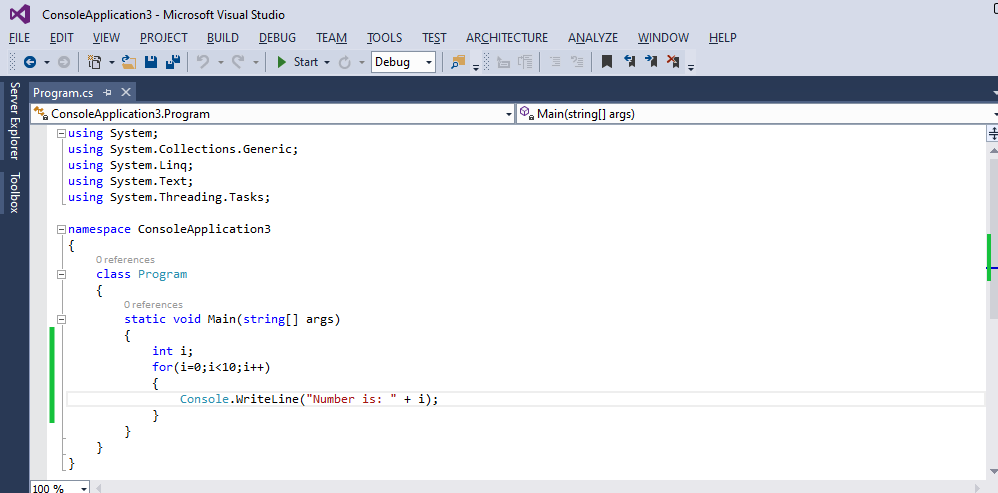# 6 Useful C# For Loop Examples

In this tutorial, i will take you through Useful C# For Loop Examples. It's often necessary to perform a sequence of logic multiple times in a program. For example, there might be a list of some items where each item needs the same processing. It's often necessary to perform a sequence of logic multiple times in a program.

For example, there might be a list of some items where each item needs the same processing. In C# there are four types of loops: the while loop, the do loop, the for loop, and the foreach loop. In this article, We will cover C# for loop only and will cover the rest of the loops in future articles.## C# For Loop Examples

### 1. Increment Operations Using For Loop

In this example program, I will show you about increment operations that you can perform using C# for loop. Here we are using default System namespaces generated by Visual Studio after selecting New Project. I have declared an int variable `i = 0` and incrementing it by 1 every time loop runs till `i < 10`. Since i has initial value 0 assigned so it will loop 10 times till `i=9` and shows the output.

```using System;
using System.Collections.Generic;
using System.Linq;
using System.Text;

namespace ConsoleApplication2
{
class Program
{
static void Main(string[] args)
{
for ( int i=0;i<10;i++)
{
Console.WriteLine("Number is: " + i);
}
}
}
}```Output### 2. Decrement Operations Using For Loop

In this example, I will show you how to perform decrement operations using C# for loop. Here we have declared `i=10` and decrementing it by 1 every time loop runs using `i--` operator till i becomes `i=1`. This loop will stop when the condition of `i>1` satisfies where the value of i cannot go less than 2.

```using System;
using System.Collections.Generic;
using System.Linq;
using System.Text;

namespace ConsoleApplication3
{
class Program
{
static void Main(string[] args)
{
int i;
for(i=10;i>1;i--)
{
Console.WriteLine("Number is: " + i);
}
}
}
}```Output### 3. Addition Operations Using For Loop

In this example I will show you addition operations using C# for loop. I have used another variable sum and initialized it to 0. I have put condition `sum +=2` which basically means `sum = sum + 2`. I am also running for loop here till `i<10` condition satisfies. So every time for loop runs it add the previous value by 2 and shows the output. This happens 10 times as you can see in the below output.

```using System;
using System.Collections.Generic;
using System.Linq;
using System.Text;

namespace ConsoleApplication3
{
class Program
{
static void Main(string[] args)
{
int i;
int sum = 0;
for(i=0;i<10;i++)
{
sum += 2;
Console.WriteLine("Sum of Number is: " + sum);
}
}
}
}```Output### 4. Subtraction Operations Using For Loop

In this example, I will show you about subtraction operations using C# for loop. Similarly, as previous example I have used a variable `sub` and initialized it to 10. Using the condition `sub -= 2` which basically means `sub = sub - 2`, every time loops runs it will decrease the output by 2.

```using System;
using System.Collections.Generic;
using System.Linq;
using System.Text;

namespace ConsoleApplication3
{
class Program
{
static void Main(string[] args)
{
int i;
int sub = 10;
for(i=0;i<10;i++)
{
sub -= 2;
Console.WriteLine("Subtraction of Number is: " + sub);
}
}
}
}```Output### 5. Multiplication Operations Using For Loop

In this example I will show you multiplication operations using C# for loop. I have used a variable mul and initialized it to 1. Here we have used the logic `mul *= 2` which means `mul = mul * 2`. You might know star(*) means multiplication operations. We are looping this logic till `i < 10` hence it will run 10 times since it starts from `i=0` as you can see in below program.

```using System;
using System.Collections.Generic;
using System.Linq;
using System.Text;

namespace ConsoleApplication3
{
class Program
{
static void Main(string[] args)
{
int i;
int mul = 1;
for(i=0;i<10;i++)
{
mul *= 2;
Console.WriteLine("Multiplication of Number is: " + mul);
}
}
}
}```Output### 6. Division Operations Using For Loop

In this example, I will show you division operations using C# for loop. Here I have initialed a variable `div = 1024` and used the logic `div /= 2` which means `div = div / 2`. So every time loop runs, it will divide the output by 2. Here also loop will run 10 times as it is started from `i=0`.

```using System;
using System.Collections.Generic;
using System.Linq;
using System.Text;

namespace ConsoleApplication3
{
class Program
{
static void Main(string[] args)
{
int i;
int div = 1024;
for(i=0;i<10;i++)
{
div /= 2;
Console.WriteLine("Division of Number is: " + div);
}
}
}
}```OutputReferences: C# Programming Guide